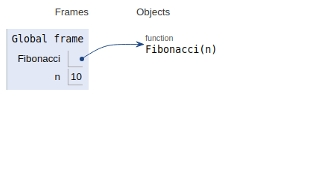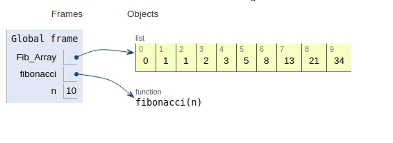# Python Program for Fibonacci numbers

PythonServer Side ProgrammingProgramming

#### Beyond Basic Programming - Intermediate Python

Most Popular

36 Lectures 3 hours

#### Practical Machine Learning using Python

Best Seller

91 Lectures 23.5 hours

#### Practical Data Science using Python

22 Lectures 6 hours

In this article, we will learn about the solution and approach to solve the given problem statement.

Problem statement −Our task to compute the nth Fibonacci number.

The sequence Fn of Fibonacci numbers is given by the recurrence relation given below

Fn = Fn-1 + Fn-2

with seed values (standard)

F0 = 0 and F1 = 1.

We have two possible solutions to the problem

• Recursive approach
• Dynamic approach

## Example

Live Demo

#recursive approach
def Fibonacci(n):
if n<0:
print("Fibbonacci can't be computed")
# First Fibonacci number
elif n==1:
return 0
# Second Fibonacci number
elif n==2:
return 1
else:
return Fibonacci(n-1)+Fibonacci(n-2)
# main
n=10
print(Fibonacci(n))

## Output

34

All the variables are declared in global scope as shown in the image below## Example

Live Demo

#dynamic approach
Fib_Array = [0,1]

def fibonacci(n):
if n<0:
print("Fibbonacci can't be computed")
elif n<=len(Fib_Array):
return Fib_Array[n-1]
else:
temp = fibonacci(n-1)+fibonacci(n-2)
Fib_Array.append(temp)
return temp
# Driver Program
n=10
print(fibonacci(n))

## Output

34

All the variables are declared in global scope as shown in the image below## Conclusion

In this article, we learnt about the approach to compute Fibonacci numbers